The nature of relativistic length shrinkage*

Danylchenko P.

SPE "GeoSystem", Vinnitsa, Ukraine

Contact with author: pavlodanylchenko@gmail.com

It is shown here, that relativistic shrinkage of the length of moving body appears itself (without the influence of external forces). This shrinkage is caused by isobaric self-contraction of body matter and by propagation of the strength of inertia forces field together with the front of body intrinsic time. A mechanism of kinetic energy filling (accumulation) of a body is considered and propagation of the phase waves of perturbation of gravitational field at supraluminal velocity is substantiated here.

## 1.Introduction

Physical processes during their separate stages can be accompanied and can be not accompanied by transfer of matter or its excited state in space. In the first case, they are characterized by group velocity V of transfer of particles and quasi-particles (photons, phonons, excitons etc.). This velocity cannot exceed the velocity of light in free space (equal to one if distances are measured in light units of length). In the second case, they are characterized by phase velocity U of propagation of change of collective space-time state of matter. The change of this state of matter realizes, as suggested here, together with the change of graviinertial stressed state in a space, filled with matter. Therefore, not only propagation of change of collective space-time state of matter but also propagation of induction of strength of inertia forces field in intrinsic frame of reference of space coordinates and time (FR) of hypothetical incompressible (perfectly rigid) body will happen momentarily (u ≡ ∞). Phase velocity of propagation of induction of spatial distribution of the strength of inertia forces field in an incompressible body, which moves relatively to the hypothetically physically homogeneous physical vacuum (PV) at a constant velocity V, in the PVFR will not be infinitely high, but equal to: U = c 2/V = V -1 (c = 1). This fact is connected with translational motion of body, in which a wave front of induction of graviinertial stressed state propagates.

## 2.Derivation of relativistic length shrinkage

Let the incompressible body moves uniformly in pseudo-Euclidean Minkowski space-time of the PVFR at absolute velocity V0 (before the induction of the strength of inertia forces field in it). Also let the initial distance along the movement route between two arbitrary points (i and j) of the body in the PVFR absolute space (in which frequency of universal background is isotropic) is equal to Xij0. Then after body transition into new steady state of its uniform motion at absolute velocity Vj = Vi = V, the distance between these two points will be equal to: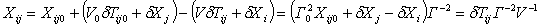, (1)

where: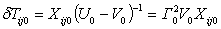(2)

and: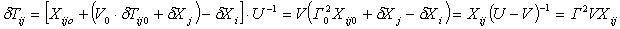(3)

- the durations of time delay of accordingly induction and removal of the strength of inertial forces field in point j, regarding point i (equal to desynchronizations, observed in the PVFR, of all the other events, which are synchronous in these points in the inertial FR (IFR) of moving body);

δXi and δXj are the traversed paths in absolute space of accordingly points i and j from the moments of induction Ti0 and Tj0 till the moments of removal Ti = Ti0 + δTi and Tj = Tj0 + δTj of strengths of inertia forces field in these points; Γ0 = (1 Ц V02)-1/2 and Γ = (1 Ц V 2)-1/2 are characteristics of the accordingly initial and newly-formed IFR;

U0 ≡ V0-1 and U ≡ V -1 - velocities of propagation of fronts of processes of induction and removal of strengths of inertia forces field in the PVFR accordingly.

LetТs assume that Xij is a function just of V and depends neither on the value of V0 nor on the law of body motion before it takes on the value of inertia motion velocity V. Then, according to (3), δTij does not depend on V0 and on this law of body motion either. Basing on this fact and on the condition V0 = 0, let's choose a uniformly accelerated motion (as the simplest law of irregular motion) of the point i of the body before it takes the value of velocity V: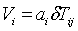, (4)

where ai = dVi /dT is the acceleration of the motion of point i. Then multiplying the left and the right parts of the equation (4) by dT and considering the immobility of the point j during the time δTijа (dX = 0), we will obtain the following differential equation: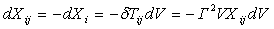, (5)

solving which we will find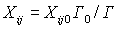,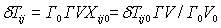. (6)

When V0:а Xij = xij / Γ, and δTij = ΓVxij,, where:а xij = Xij (0) is the distance between the points j and i, measured in the IFR of moving body and equal to the distance between them in absolute space in hypothetical state of body absolute rest relatively to the PV.

So, if an incompressible body proceeds from the state of rest relatively to the PV into a new-steady state of inertial motion, then relativistic shrinkage of body length along the direction of motion takes place. The value of this shrinkage is determined by the analytical dependence, discovered by Fitzgerald and Lorentz independently from each other, and does not depend on the law of change of strengths: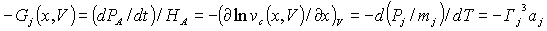of removable gravitational (graviinertial) field, which originates in the intrinsic FR of the body. And consequently, the value of this shrinkage does not depend on the law of motion of body points during the proceeding of body from the state of rest or inertial motion into the state of inertial motion at another velocity. Here: PA and HA - accordingly linear momentum and invariable energy (conservative Hamiltonian) of free-falling (motionless in the PVFR) particle A, which are definite in intrinsic FR of the accelerating body;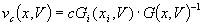а- improper values of the velocity of light in free space in body intrinsic FR (unequal in different points of physically inhomogeneous intrinsic space of body in proper quantum time t of point i);аа Pj and mj - accordingly linear momentum in the PVFR and eigenvalue of mass of the point object (particle) j of the body. At this, conditions: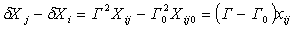,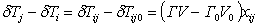, (7)

which follow from (1-3), always guarantee simultaneity of removal of strengths of inertial forces field in body intrinsic FR in all body points. And consequently, they also guarantee a momentary transition in this FR (without any transient process) of an incompressible body into equilibrium state of inertial motion. And the fulfillment of these conditions is possible only at the following distribution of strength of inertia forces field along the moving body: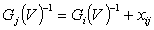. (8)

Where, as we supposed, Gi(V) can vary with time according to the arbitrary law. And it specifies at that any law of body motion. At that spatial distribution of strength of graviinertial field (inertia forces field) unconditional realization of the identity will take place: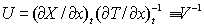.

## 3. Equations of irregular motion of body points

According to (8), the motion of any point of the body in the process of its transition from inertial motion at absolute velocity V0 to inertial motion at absolute velocity V can be described by the same parametric equations as motion of the point i: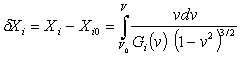, (9)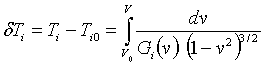, (10)

or in another form by the equation: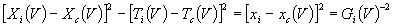(11)

Where: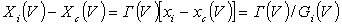, (12)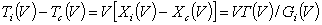, (13)

and: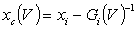(14)

is a coordinate of an asymptotic limit (singular plane) of intrinsic space of moving body, which can be considered as an observer horizon of the body FR (Gc = );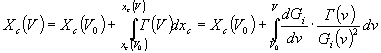, (15)

is a coordinate in absolute space of hypothetically initial position of the observer horizon of body at the beginning of its motion (V = 0) on condition that distribution of strengths of inertia forces field along the body from the beginning of its motion is the same as at the given identical velocity of all its points Gi(0) = Gi(V) = const(V);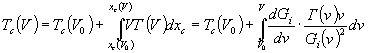, (16)

is a hypothetical moment of absolute time, when the body motion in the absolute PV space would begin, if distribution of strengths of inertia forces field along the body were stationary. At that, Tc(0) = Ti(0). And at coincidence of the points of origin in absolute space and in intrinsic space of the body in its hypothetic state of rest relatively to the PV (Xi(0) = xi(0)) and also Xc(0) = xc(0). When dependence of strengths of inertia forces field on absolute velocities V of body points is weak, equation (11) corresponds to quasi-hyperbolic motion of these points. If the distribution of strengths of inertia forces field along the moving body is stationary (Gi  const (V)), then all the body points perform in the PVFR not quasi-hyperbolic but definitely a hyperbolic motion. And the moving body (even if it is compressible) will be resting in an appropriate to it Möller rigid accelerating FR [1,2]. A proportional mutual synchronization of quantum clock, located in different points of physically inhomogeneous intrinsic space of this FR, is possible only in this FR. But in general case, events in different points are considered just to coincide with each other, if they happen at the same instantaneous values of absolute velocities V of these points. Events, not having direct cause-and-effect relations with each other, are meant here under coincident events. But in the presence of common cause, mutual correlation of coincident events can take place. These events correspond to definite collective space-time state (microphase state) of particles of body matter. And they are simultaneous according to quantum clock, only in the case of homogeneity of intrinsic time t, which is possible only in the case of stationarity of spatial distribution of the velocity of light in free space in co-moving FR: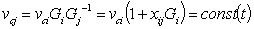.

And it is possible only in Möller FR [1, 2].

From the condition of the absence of increment of action S: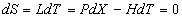, corresponding to invariance of collective space-time state of matter, we will have: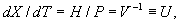where L - Lagrangian of body matter. In view of this, the front of induction of strength of inertia forces field in incompressible body (the same as the front of propagation of change of collective space-time state of matter) is identical to the front of coincident events.

According to Lorentz transformations, the front of coincident events of any body, which moves relatively to an observer at velocity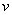, will be moving in observer FR, as well as in the PVFR, not at infinitely high, but at finite phase velocity u = vc2/v.

## 4. Propagation of the changes of graviinertial stressed state and elastic deformation in compressible bodyа

For an elastically compressible (deformable) body, the distance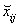аbetween the points i and j in its uniform and stable metrical intrinsic space (where the motion of its points in the process of elastic deformation of the body matter is observable) can be connected with the distance between them xij in inseparable from the body its nonuniform and metrically instable physical intrinsic space by the following dependence: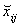= α(V)xij. Where: α(V) is a coefficient of the elastic shrinkage of body size along the direction of its motion, that depends on the velocity of the body when the strength of inertia forces field is instable (G ≠  const(V)).

аIn contrast to a hypothetical incompressible body, in metrical intrinsic space of a compressible body, only microobjects (elementary particles) have purely relativistic size shrinkage along the direction of motion. It is connected with the elastic deformation of matter macroobjects that also can be observed in the body intrinsic FR. As well as in incompressible body, this shrinkage is caused by elementary particles adaptation (and by adaptation of the matter as the whole, owing to Van der Waals forces, which have electromagnetic nature) to changed conditions of elementary particles interaction. This adaptation guarantees in the first place the isotropy of interaction frequency and becomes apparent in co-moving FR at the absence of anisotropy of the radiation spectrum of radiation sources, motionless relatively to the body. Lorentz considered processes, which are connected with this adaptation, first in detail  on the example of electrical and electromagnetic phenomena. The possibility of such adaptation follows from the wave nature of elementary particles and of matter as the whole. ThatТs why relativistic size shrinkage realizes at elementary particles level and is connected with longitudinal self-contraction (which is initiated by motion) of wave-like formations, which correspond to elementary particles .

Relativistic size shrinkage along the direction of the body motion will guarantee isotropy of the velocity of light in free space only in physical intrinsic space of the compressible body. In metrical intrinsic space (considering the observability of body deformation in it) the velocity of light in free space will be anisotropic. Moreover, in contrast to physical intrinsic space, in metrical intrinsic space definition of an interval between two world points (which is invariant to transformations of coordinates only in physical spaces), as well as the definition of energy and momentum of any objects has no physical sense. That is why the analysis of dynamics of body compression and of the motion of objects is impossible in principle in this space . Dynamics of the objects can be analyzed only in physical intrinsic space of a compressed body using continuous renormalization of all the measurements and spatial characteristics, determined in it, considering their change in metrical intrinsic space of the body.

In elastic compressible body, as well as in hypothetical incompressible body, the front of induction of strengths of graviinertial field in it can be identified with a wave front of change of collective space-time state of body matter. That is why, also in an intrinsic FR of elastically compressible body the propagation not only of quantum of action, but also of change of space-time distribution of the strength of graviinertial field (the main characteristic of collective space-time state of matter), takes place momentarily in principle. This is caused by the wave nature of elementary particles of matter, which becomes apparent also in collective space-time state of matter.

Let the linear momentum and therefore velocity of an elastic compressible body increase in a result of a shock action (impact). Then in the PVFR (as well as in FR of any body that moves at another velocity) a phase soliton (phase packet) of modulation of strength of graviinertial field will run along the moving body at supraluminal phase velocity. This soliton changes the value of relativistic shrinkage of molecules of body matter and does not cause their elastic deformation. After that, a soliton (wave packet) of elastic deformation and excitation of matter molecules will run along the body at a velocity of sound. Body filling with additional kinetic energy, as it were transferred by graviinertial phase soliton at supraluminal velocity, as a matter of fact is not connected with ripple-through energy transfer in it. This filling is an inert process and realizes only due to accumulation of difference of Doppler energies of exchange virtual elementary particles and quasi-particles, which propagate in the process of interaction of elementary particles, atoms and molecules in and against the direction of soliton propagation. These are virtual pi-mesons that during the process of strong interaction maintain collective dynamic equilibrium between protons and neutrons in an atom. And these also are virtual photons that during the process of electromagnetic interaction maintain collective dynamic equilibrium between protons and electrons in an atom, as well as between electrically and magnetically polarized atoms and molecules. At this, the work is executed by the forces, which disturb the mechanical equilibrium of the matter. These forces are equal to inertia forces (they are, like gravitational forces, actually only pseudoforces ), but they are directed oppositely. Neither entropy nor enthalpy of the matter in the body intrinsic FR vary during the process of the body filling with kinetic energy. That is why neither free photons nor phonons or any other quasi-particles are generated and transferred in the body. And therefore, ripple-through energy transfer and energy dissipation are absent. In the contrast to total energy, Helmholtz energy of body matter increases in the PVFR not only due to increase of linear momentum and of relativistic shrinkage of molecular volume, but also due to decrease of Planck relativistic temperature. The energy of soliton of elastic deformation (which runs after the phase soliton) will be dissipated and transformed into heat after multiple reflections from the body boundaries.

In this case, before coming of a soliton of elastic deformation of matter, motion of points of compressible body can be described by the same equations (8-16) as motion of points of incompressible body. Now let the body linear momentum increase due to a long-lived force. And thatТs why the front of removal of the graviinertial stressed state runs along the body after the induction of a definite elastic deformation of its matter. Then motion of the body points before the front running can be described by equations, different from the ones (8-16). The distance between the points j and i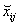аin elastically deformated state of the body matter will be used in these equations instead of the distance xij. In this case, removal of graviinertial stressed state will realize the same way as in an incompressible body, but considering the change of the distances xij to distances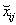аthat take place at a point in time when the front of removal of the stressed state is passing through the point j.

Relativistic shrinkage of the length of moving body realizes itself (without influence of any external forces) and its matter does not exhibit resistance to contraction. Therefore work on relativistic contraction of matter: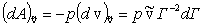,

executes by internal forces due to decrease of heat content (enthalpy) of moving body matter: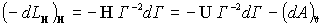,

where: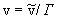and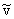- accordingly relativistic value and eigenvalue of matter molar volume;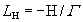- Lagrangian of enthalpy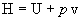, and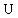а- internal energy of the one mole of matter. At that the energy of thermal oscillatory motion of matter molecules partially transforms into kinetic energy of their ordered motion.

## 5. Effects caused by equivalence of removable (graviinertial) and irremovable gravitational fields

Momentary propagation of induction of graviinertial stressed state (physical inhomogeneity of the body intrinsic space, that can be identified to a gravitational field) in the body is well matched in Einstein-Podolski-Rosen paradox [6,7] with a momentary intercoordination of changes in the quantum-mechanical characteristics of previously correlated photons or elementary particles after mutual self-distancing of the ones at arbitrary long spacing. This points at the possibility of momentary propagation of disturbance of proper gravitational field (in intrinsic FR) also in free space. Also this points at rigid connection in space of gravitational field to macro- or microobject, which creates it, (at the impossibility of time delay of displacement of spatial distribution of gravitational field strength relatively to displacement of this object). That is why the carriers of gravitational field in free space are photons, elementary particles and any moving macroobjects, consisting of them, but not hypothetical gravitons (their existence is impossible in principle as it was shown in ). Any moving macroobject (body) can be characterized by de Broglie frequency. And it lets us consider moving body also as a Уgraviton waveФ, which transfers energy.а

During the rotation of a negatively charged body around a positively charged body, electromagnetic radiation is generated. But similar specific radiation is not generated during the rotation of planets around the Sun. Otherwise the planets finally would fall on the Sun due to continuous energy losses. That is why only the matter, the accretion of which happens from one star to another in a compact system of double star, can be considered as Уgraviton radiationФ.

Phase gravitational waves (caused, for example, by the rotation of astronomical body around the point, which is not coincide with its centre of mass) that propagate in free space at supraluminal velocity give rise to disturbance in the motion of another astronomical bodies, not executing any work. At this, only transition of internal energy of the matter of astronomical bodies into kinetic energy realizes, as it does during a free fall of macroobjects in terrestrial gravitational field. These phase waves are progressive waves of metrical and physical microinhomogeneities of space in the form of space-time modulations of permittivity and permeability of PV, which uniquely determine metrical and physical properties of the space, filled with PV. Space-time modulations of permittivity and permeability of PV also appear in the process of propagation of radiation because of the presence of negative feedbacks, which guarantee self-restriction of improper values of electric and magnetic strengths of electromagnetic wave. And these negative feedbacks also cause the formation of wave packets of quanta of electromagnetic energy (soliton-like photons) nearby elementary particles because of the presence of very significant metrical and physical microinhomogeneities of space in their neighbourhood.

## 6. Conclusions

The relativistic shrinkage of the length of moving body is purely kinematics effect. This effect is connected with the change of relativistic values of matter thermodynamic parameters and not connected with matter elastic properties. At any law of body motion this shrinkage appears and varies during the process of isobaric self-contraction of matter. This process always passes ahead of the process of change of matter elastic compression. And this is caused by propagation of changes of the strength of inertia forces field together with propagation of changes of collective space-time state of matter (propagation of front of body intrinsic time).

Phase waves of perturbation of removable (graviinertial) and irremovable gravitational fields donТt transfer energy themselves. They only create necessary conditions for energy transfer in the process of the interaction of matter elementary particles. This energy transfer realizes due to accumulation of difference of Doppler values of energies of exchange virtual elementary particles and quasi-particles, which propagate in and against the direction of the body motion. ThatТs why these waves can propagate at supraluminal velocity.

### Reference list

. а Möller C., The Theory of Relativity, Clarendon Press Oxford, 1972

. а Danylchenko P.,а Phenomenological justification of relativistic shrinkage of the length of moving body, in: The Gauge-evolutional theory of the Creation, Ukraine, Vinnytsia, 1994, 1, 5

. а Lorentz H., The theory of electrons, Leipzig: Teubner, 1916

. а Danylchenko P., Foundations of the Gauge-evolutional theory of the Creation (space, time, gravitation and the Universe expansion), Ukraine,Vinnytsia,1994

. а Danylchenko P., Nonrigid frames of reference contracting in Minkowski space-time, in: The Gauge-evolutional theory of the Creation, Ukraine, Vinnytsia, 1994, 1, 52

. а Einstein A., Podolski B.,а Rosen N., Philos. Rev., 1935, 47, 7

.аа Vigier J.-P., paper on Einstein-Podolski-Rosen paradox, in Einstein Centenarium, ed. H.-J. Treder Berlin: Akademie Verlag, 1982

*а Supplemented and completed version of the article from the collection of articles УGauge-Evolutional Interpretation of Special and General RelativitiesФ, Vinnitsa, O. Vlasuk, 2004

Хостинг от uCoz